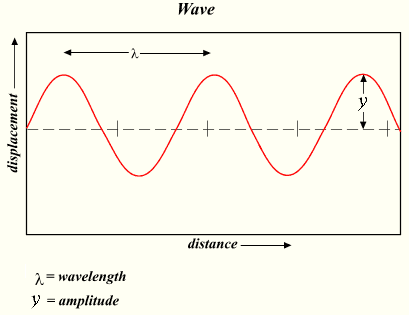# Wavelength Calculator

The calculator requires you to input any two of the following three parameters: wave speed, frequency (or wave period) and wavelength. Once you input two parameters, the calculator will calculate the remaining one and display the result.

1. Wavelength: The wavelength is the distance between two consecutive points on a wave that are in phase, such as the distance between two consecutive peaks or two consecutive troughs. It is usually represented by the Greek letter lambda (λ) and is measured in meters (m), centimeters (cm), or nanometers (nm), depending on the type of wave.
2. Period: the time it takes for one complete cycle of a wave to occur. It is typically denoted by the letter T and is measured in units of time, such as seconds or milliseconds. The period and frequency of a wave are inversely related, meaning that as the period increases, the frequency decreases, and vice versa.
3. Frequency: the number of complete cycles of a wave that occur in a given unit of time. It is typically denoted by the letter f or ν (nu) and is measured in units of hertz (Hz), which represents one cycle per second. The frequency and period of a wave are inversely related, meaning that as the frequency increases, the period decreases, and vice versa.
4. Wave velocity: The wave velocity is the speed at which a wave travels through a medium. It is usually represented by the symbol v and is measured in meters per second (m/s). The wave velocity is determined by the properties of the medium through which the wave is traveling, such as the density, elasticity, and temperature of the medium.These parameters are related to each other through the wave speed equation: . This equation states that the speed at which a wave travels through a medium is equal to the product of its wavelength and frequency. Therefore, if you know the wavelength and frequency of a wave, you can calculate its speed. Conversely, if you know the speed and frequency of a wave, you can determine its wavelength, and if you know the speed and wavelength, you can find the frequency.

For example, if you input the wave speed and frequency, the calculator will use the formula to calculate the wavelength. Similarly, if you input the wave period and wave speed, the calculator will use the formula to calculate the wavelength.#### Traveling Wave Relationship

Digits after the decimal point: 3
Velocity, m/s

Wavelength, m

Period, s

Frequency, Hz

URL copiado para a área de transferência
PLANETCALC, Wavelength Calculator